# 基于三个典型函数的交叉熵优化方法评价研究Research on the Cross Entropy Optimization Method Evaluation Based on the Three Typical Functions

• 全文下载: PDF(611KB)    PP.94-99   DOI: 10.12677/AAM.2020.91011
• 下载量: 44  浏览量: 88

The cross entropy optimization method is transformed from the cross entropy method to estimate the probability of failure. By constructing the artificial reliability problem, it concentrates the problem on the search of the maximum value, and then the minimum value of the function can be solved. According to three typical functions, the test results of the cross entropy optimization method are compared with those of the genetic algorithm. The results show that the global convergence rate and accuracy of the cross entropy optimization method are better than the genetic algorithm for single-peak and multi-peak functions. For this Pathological function, which is difficult to minimize globally, the cross entropy optimization method also overcomes the problem of local optimization, and the optimization result is closer to the theoretical optimal value.

1. 引言

2. 原理

$\mathrm{min}s\left(x\right)\text{\hspace{0.17em}}\text{\hspace{0.17em}}\text{\hspace{0.17em}}\text{\hspace{0.17em}}\text{\hspace{0.17em}}{x}^{L}\le x\le {x}^{U}$ (1)(2)

${P}_{f}={\int }_{s\left(x\right)\le s}{f}_{X}\left(x;v\right)\text{d}x=E\left[{I}_{s\left(x\right)\le {s}^{\ast }}\right]$ (3)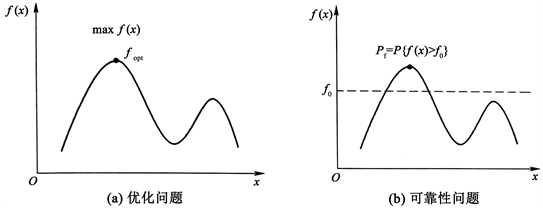Figure 1. Similarities between optimization problems and reliability problems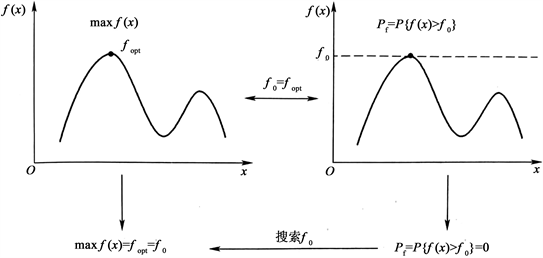Figure 2. The transition between optimization problems and reliability problems

3. 方法步骤

1) 初始化待定分布参数 ${v}_{0}=\theta$，迭代记数 $k=0$

2) 从 ${f}_{X}\left(x;{v}_{k}\right)$ 产生N个随机样本 $\left\{{x}_{1},\cdots ,{x}_{N}\right\}$，代入函数中求值 $\left\{s\left({x}_{i}\right)\right\}$ 并排序，依据式 ${b}_{k}={s}_{\left[\rho N\right]}$ 确定的值，其中 ${b}_{k}$ 为功能函数次序统计量的 $\rho$ 分位数， $\left\{{s}_{i}=s\left({x}_{i}\right)\right\}$ 为从小到大排序后的统计量，即 ${s}_{1}$ 为N个样本中最小值， ${s}_{N}$ 为N个样本中最大值。

3) 使用同样的N个随机样本 $\left\{{x}_{1},\cdots ,{x}_{N}\right\}$，解式 $\mathrm{max}\frac{1}{N}\underset{i=1}{\overset{N}{\sum }}{I}_{\left\{s\left(x\right)\le {b}_{k}\right\}}\left({x}_{i}\right)\mathrm{ln}\left({f}_{X}\left({x}_{i};{v}_{k-1}\right)\right)$，设权函数，从而得 ${v}_{k+1}$ 新的参数的估计值 ${\stackrel{^}{v}}_{k+1}$

4) 若达到某种收敛准则，算法停止，否则设 $k=k+1$，转步骤(2)。

4. 实验分析

${f}_{1}\left(x\right)=\underset{i=1}{\overset{10}{\sum }}{x}_{i}^{2},\text{\hspace{0.17em}}\text{\hspace{0.17em}}\text{\hspace{0.17em}}-100\le {x}_{i}\le 100,\text{\hspace{0.17em}}\text{\hspace{0.17em}}i=1,2,\cdots ,10$ (4)

${f}_{2}\left(x\right)=0.5+\frac{\mathrm{sin}\sqrt{{x}_{1}^{2}+{x}_{2}^{2}}-0.5}{{\left[1+0.001\ast \left({x}_{1}^{2}+{x}_{2}^{2}\right)\right]}^{2}},\text{\hspace{0.17em}}\text{\hspace{0.17em}}\text{\hspace{0.17em}}-100\le {x}_{1},{x}_{2}\le 100$ (5)

${f}_{3}\left(x\right)=\underset{i=1}{\overset{9}{\sum }}\left[100\ast {\left({x}_{i+1}-{x}_{i}^{2}\right)}^{2}+{\left({x}_{i}-1\right)}^{2}\right],\text{\hspace{0.17em}}\text{\hspace{0.17em}}\text{\hspace{0.17em}}-100\le {x}_{i}\le 100,\text{\hspace{0.17em}}\text{\hspace{0.17em}}i=1,2,\cdots ,9$ (6)Table 1. The average optimal value of a function that runs 20 times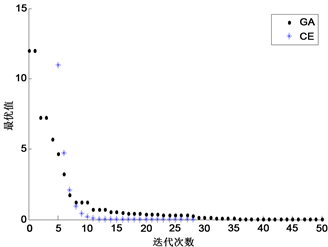Figure 3. The optimal value iteration curve of ${f}_{1}\left(x\right)$ function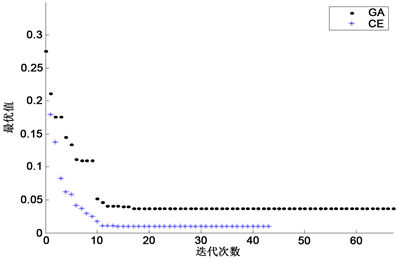Figure 4. The optimal value iteration curve of ${f}_{2}\left(x\right)$ function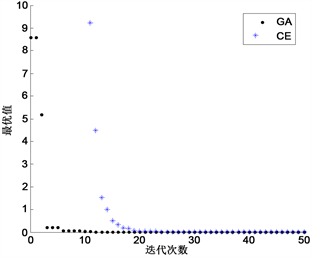Figure 5. The optimal value iteration curve of ${f}_{3}\left(x\right)$ function

5. 结论

  Rubinstein, R. (1999) The Cross-Entropy Method for Combinatorial and Continuous Optimization. Methodology & Computing in Applied Probability, 1, 127-190. https://doi.org/10.1023/A:1010091220143  Helvik, B.E. and Wittner, O. (2001) Using the Cross-Entropy Method to Guide/Govern Mobile Agent’s Path Finding in Networks. In: Pierre, S. and Glitho, R., Eds., Mobile Agents for Telecommunication Applications, Springer, Berlin, Heidelberg, 255-268. https://doi.org/10.1007/3-540-44651-6_24  Alon, G., Kroese, D.P., Raviv, T., et al. (2005) Application of the Cross-Entropy Method to the Buffer Allocation Problem in a Simulation-Based Environment. Annals of Operations Research, 134, 137-151. https://doi.org/10.1007/s10479-005-5728-8  Ghidey, H. (2015) Reliability-Based Design Optimization with Cross-Entropy Method. Ph.D. Thesis, Norwegian University of Science and Technology, Norway.  Chepuri, K. and Homem-De-Mello, T. (2005) Solving the Vehicle Routing Problem with Stochastic Demands Using the Cross-Entropy Method. Annals of Operations Research, 134, 153-181. https://doi.org/10.1007/s10479-005-5729-7  Kroese, D.P., Porotsky, S. and Rubinstein, R.Y. (2006) The Cross-Entropy Method for Continuous Multi-Extremal Optimization. Methodology and Computing in Applied Probability, 8, 383-407. https://doi.org/10.1007/s11009-006-9753-0  任超, 张航, 李洪双. 随机优化的改进交叉熵方法[J]. 北京航空航天大学学报, 2018, 44(1): 205-214.